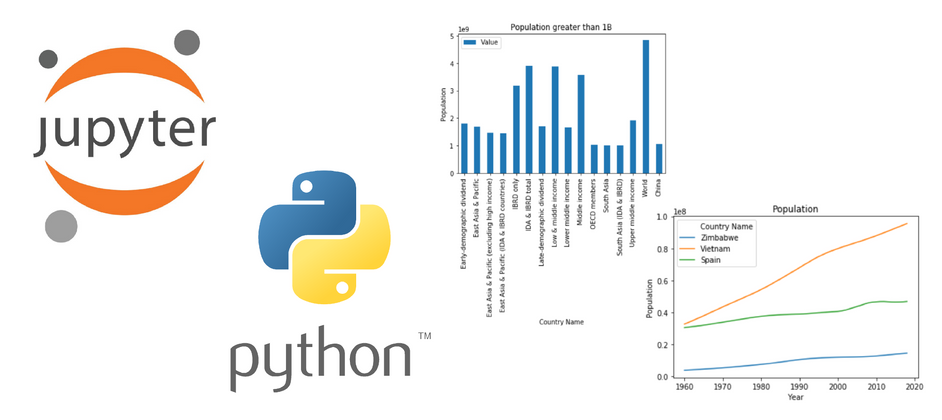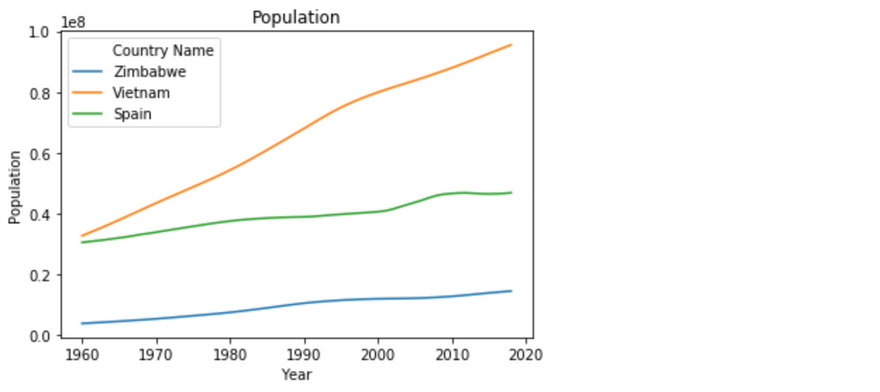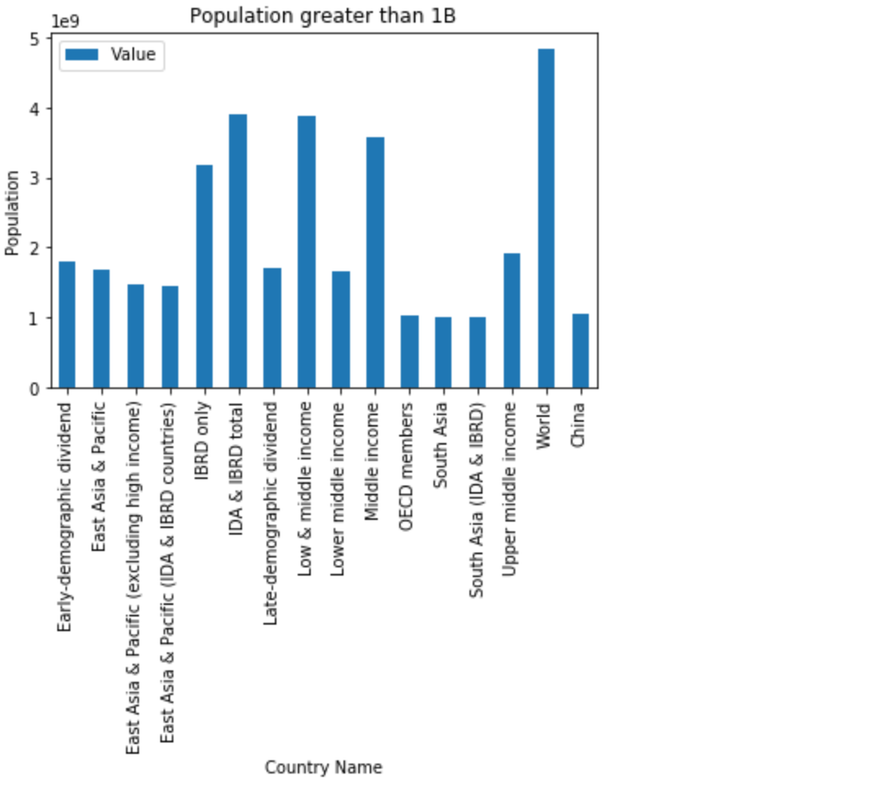## CodeNewbie Community is a community of 8,699 amazing newbies# Data Analysis in Python using Jupyter Notebook - Part 2

In this tutorial we will expand on our knowledge and learn how to plot multiple lines on the same graph, streamline our code, and create bar graphs.

## Preliminary Steps

If you have not worked through Part 1, to ensure you're able to run the code in this tutorial, make sure to obtain the population data set and run the following based on where you have saved the file:

``````import matplotlib.pyplot as plt
import pandas as pd
``````

## Explore the data

To further understand our data set and know the unique countries/regions in the data, we'll use the following line of code. This will give us a table with only one country/region per row so we can see all the places listed.

``````popData.drop_duplicates(subset = "Country Name")
``````

We can see there are 263 unique parts of the world listed after running the above code.

## Plotting Multiple Lines on the Same Plot

Now we'll try plotting multiple countries on the same graph to see how their populations grow relative to each other. We can filter the countries we want to plot using the 'Country Name' field.

``````pop = popData.loc[(popData['Country Name'] == 'Zimbabwe') |
(popData['Country Name'] == 'Vietnam') |
(popData['Country Name'] == 'Spain')]
``````

I initially struggled to figure out how to put these three countries on the same plot. After some research, I learned that there is one line of code using the Seaborn package that accomplishes this. Next, we’ll add some labels to the graph to make it clear.

``````import seaborn as sns
sns.lineplot(data = pop, x = 'Year', y = 'Value', hue = 'Country Name')
ylab = 'Population'
title = 'Population'
plt.ylabel(ylab)
plt.title(title)
``````

While we can see that all this code works well, there is a more streamlined way we can obtain the data for specific countries. This can be done by changing the indices of the rows of the table. With the below line, now the country code is the index.

``````popData.index = popData['Country Code']
``````

As you can see, the numerical index for each row has now been replaced with the Country Code.

``````popData.head
``````

Now using .loc with the Country Code, the data for a specific country or region can be obtained.

``````popData.loc['ZWE']
``````

To avoid the redundancy of the country code getting listed twice, the following code removes the display of the index.

``````zwe = popData.loc['ZWE']
print(zwe.to_string(index = False))
``````

Now we can continue to plot the data for Zimbabwe just as we did in Part 1 of this series.

``````zwe.plot('Year', 'Value')
ylab = 'Population'
title = 'Zimbabwe Population'
plt.ylabel(ylab)
plt.title(title)
``````

Writing zwe = popData.loc['ZWE'] is much more steamlined than zwe = popData.loc[popData['Country Name'] == 'Zimbabwe']. Before the code had to check whether the field ‘Country Name’ was equal to Zimbabwe. With the current code, it only needs to check whether the index value is ‘ZWE’. Now we can use this same idea to rewrite our code for plotting Spain, Zimbabwe, and Vietman on the same graph!

``````pop = popData.loc[['ZWE', 'VNM', 'ESP']]
sns.lineplot(data = pop, x = 'Year', y = 'Value', hue = 'Country Name')
ylab = 'Population'
title = 'Population'
plt.ylabel(ylab)
plt.title(title)
``````

As we can see, we’re able to get a line plot with all three countries just like we did before.## Filtering by Population Value

Now we are going to display a bar graph with data that satisfies the conditions below.

``````highPop = popData.loc[(popData.Value > 1000000000) &
(popData.Year == 1985)]
``````

For plotting a bar graph, we can use the ‘kind’ field to denote a bar graph.

``````highPop.plot('Country Name', 'Value', kind = "bar")
ylab = 'Population'
title = 'Population > 1B'
plt.ylabel(ylab)
plt.title(title)
``````

We get a bar graph with all the countries/regions that satisfy our criteria.To filter the years where Zimbabwe has a population is over 10000000, we can do the following:

``````zwePop = popData.loc[(popData['Country Name'] == 'Zimbabwe') &
(popData.Value > 10000000)]
zwePop.plot('Year', 'Value', kind = "bar")
ylab = 'Population'
title = 'Zimbabwe Population'
plt.ylabel(ylab)
plt.title(title)
``````

## Up Next

Now you have learned a little more on plots. We'll continue building upon what we've learned here in the next installment!